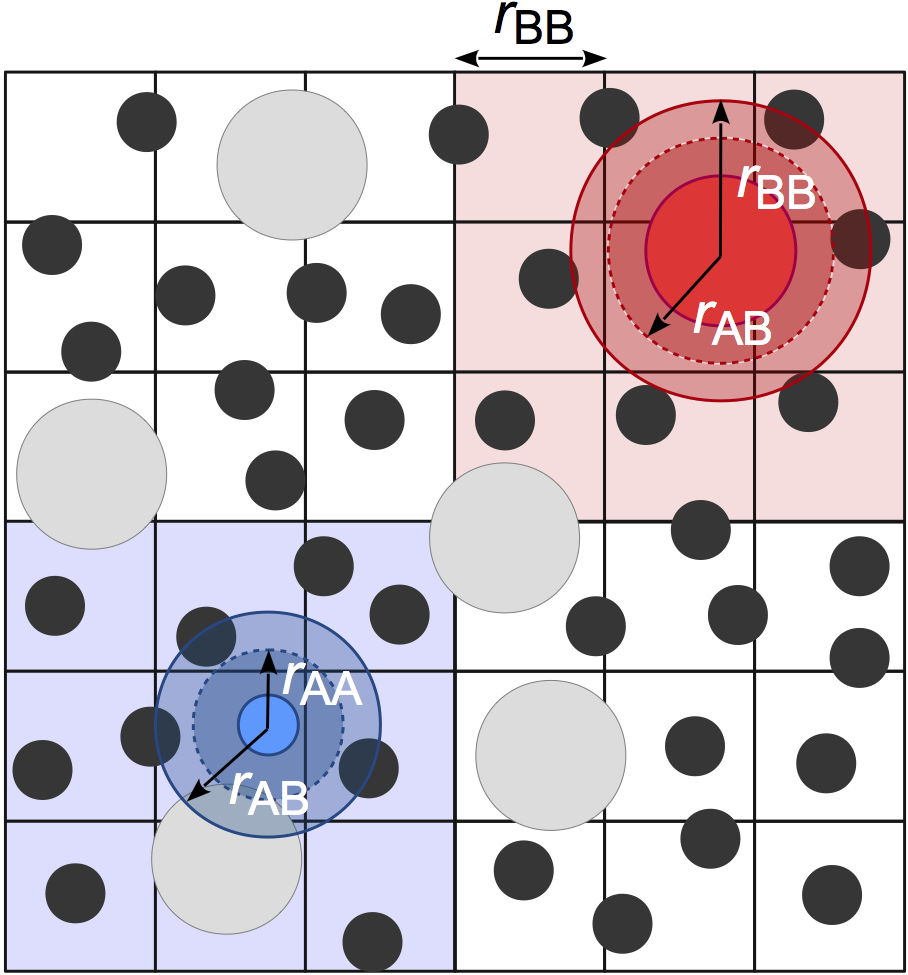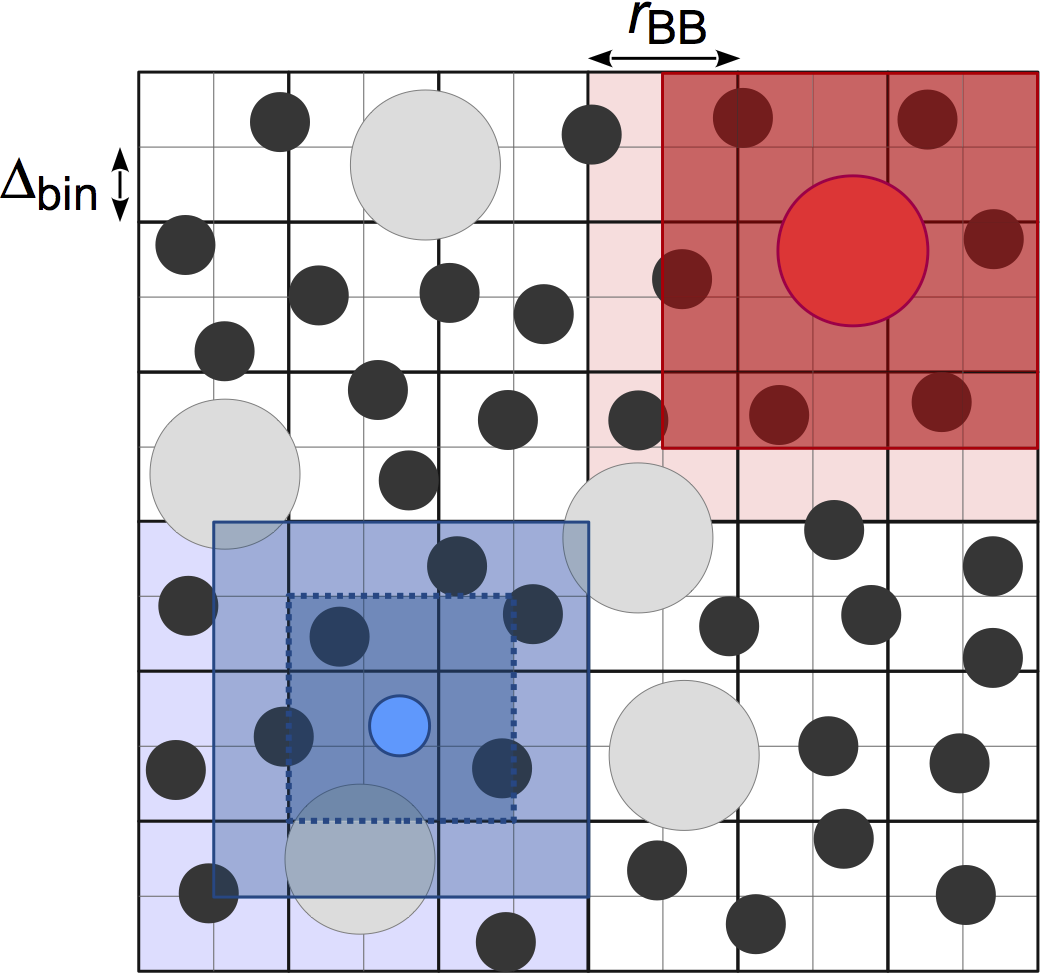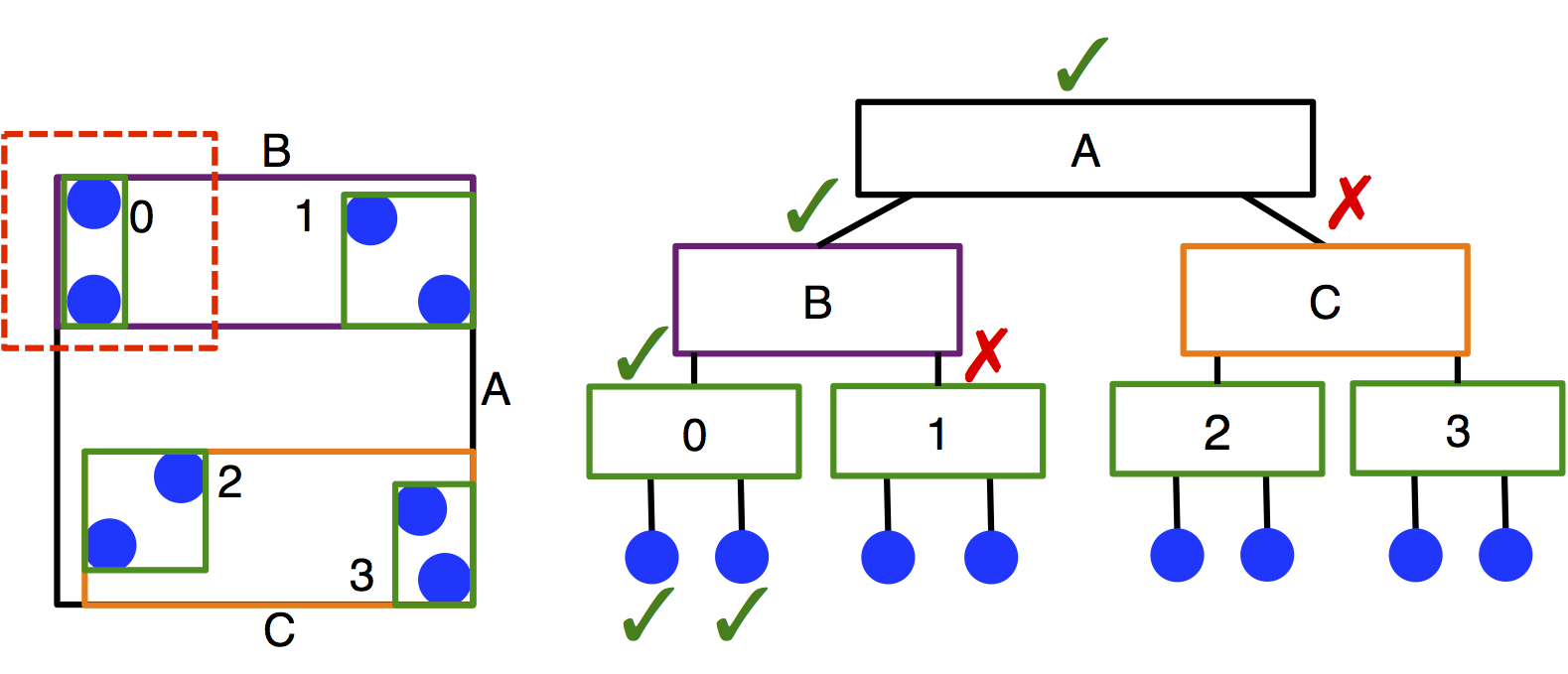# md.nlist¶

Overview

 NeighborList Base class neighbor list. Cell Neighbor list computed via a cell list. Stencil Cell list based neighbor list using stencils. Tree Bounding volume hierarchy based neighbor list.

Details

Neighbor list acceleration structures.

Pair forces (hoomd.md.pair) use neighbor list data structures to find neighboring particle pairs (those within a distance of $$r_\mathrm{cut}$$) efficiently. HOOMD-blue provides a several types of neighbor list construction algorithms that you can select from: Cell, Tree, and Stencil.

Multiple pair force objects can share a single neighbor list, or use independent neighbor list objects. When neighbor lists are shared, they find neighbors within the the maximum $$r_{\mathrm{cut},i,j}$$ over the associated pair potentials.

Buffer distance

Set the NeighborList.buffer distance to amortize the cost of the neighbor list build. When buffer > 0, a neighbor list computed on one step can be reused on subsequent steps until a particle moves a distance buffer/2. When NeighborList.check_dist is True, NeighborList starts checking how far particles have moved NeighborList.rebuild_check_delay time steps after the last build and performs a rebuild when any particle has moved a distance buffer/2. When NeighborList.check_dist is False, NeighborList always rebuilds after NeighborList.rebuild_check_delay time steps.

Note

With the default settings (check_dist=True and rebuild_check_delay=1), changing NeighborList.buffer only impacts simulation performance and not correctness.

Set the buffer too small and the neighbor list will need to be updated often, slowing simulation performance. Set the buffer too large, and hoomd.md.pair.Pair will need to needlessly calculate many non-interacting particle pairs and slow the simulation. There is an optimal value for NeighborList.buffer between the two extremes that provides the best performance.

Base distance cutoff

The NeighborList.r_cut attribute can be used to set the base cutoff distance for neighbor list queries. The actual cutoff distance is always the maximum $$r_{\mathrm{cut},i,j}$$ of the base cutoff and associated pair potentials.

Note

This attribute is particularly useful for implementing custom pair forces in Python.

Attention

Users should only set this attribute when utilizing the accessor APIs, pair_list, local_pair_list, cpu_local_nlist_arrays, or gpu_local_nlist_arrays.

Exclusions

Neighbor lists nominally include all particles within the chosen cutoff distances. The NeighborList.exclusions attribute defines which particles will be excluded from the list, even if they are within the cutoff. NeighborList.exclusions is a tuple of strings that enable one more more types of exclusions. The valid exclusion types are:

• 'angle': Exclude the first and third particles in each angle.

• 'body': Exclude particles that belong to the same rigid body.

• 'bond': Exclude particles that are directly bonded together.

• 'meshbond': Exclude particles that are bonded together via a mesh.

• 'constraint': Exclude particles that have a distance constraint applied between them.

• 'dihedral': Exclude the first and fourth particles in each dihedral.

• 'special_pair': Exclude particles that are part of a special pair.

• '1-3': Exclude particles i and k whenever there is a bond (i,j) and a bond (j,k).

• '1-4': Exclude particles i and m whenever there are bonds (i,j), (j,k), and (k,m).

class hoomd.md.nlist.NeighborList(buffer, exclusions, rebuild_check_delay, check_dist, mesh, default_r_cut)

Base class neighbor list.

NeighborList is the base class for all neighbor lists.

Warning

Users should not instantiate this class directly. The class can be used for isinstance or issubclass checks.

buffer

Buffer width $$[\mathrm{length}]$$.

Type

float

exclusions

Defines which particles to exclude from the neighbor list, see more details above.

Type

tuple[str]

rebuild_check_delay

How often to attempt to rebuild the neighbor list.

Type

int

check_dist

Flag to enable / disable distance checking.

Type

bool

mesh

mesh data structure (optional)

Type

Mesh

default_r_cut

Default cutoff distance $$[\mathrm{length}]$$ (optional).

Type

float

r_cut

Base cutoff radius for neighbor list queries. $$[\mathrm{length}]$$. Optional: defaults to the value default_r_cut specified on construction.

Type: TypeParameter [tuple [particle_type, particle_type], float])

property cpu_local_nlist_arrays

Expose nlist arrays on the CPU.

Provides direct acces to the neighbor list arrays on the cpu. All data is MPI rank-local.

The hoomd.md.data.NeighborListLocalAccess object exposes the internal data representation to efficently iterate over the neighbor list.

Note

The local arrays are read only.

Examples:

with self.cpu_local_nlist_arrays as arrays:
for i, (head, nn) in enumerate(nlist_iter):
j = arrays.nlist[j_idx]
# i and j are "neighbor" indices

Type

hoomd.md.data.NeighborListLocalAccess

property gpu_local_nlist_arrays

Expose nlist arrays on the GPU.

Provides direct access to the neighbor list arrays on the gpu. All data is MPI rank-local.

The hoomd.md.data.NeighborListLocalAccessGPU object exposes the internal data representation to efficently iterate over the neighbor list.

Note

The local arrays are read only.

Examples:

get_local_pairs = cupy.RawKernel(r'''
extern "C" __global__
void get_local_pairs(
const unsigned int N,
const unsigned int* nns,
const unsigned int* nlist,
const unsigned int* tags,
const unsigned long* offsets,
unsigned long* pairs) {
unsigned int i = (unsigned int)
if (i >= N)
return;
uint2* pair = (uint2*)pairs;
unsigned int nn = nns[i];
unsigned long offset = offsets[i];
unsigned int tag_i = tags[i];
for (unsigned int idx = 0; idx < nn; idx++) {
unsigned int j = nlist[head + idx];
pair[offset + idx] = make_uint2(tag_i, tags[j]);
}
}
''', 'get_local_pairs')

with nlist.gpu_local_nlist_arrays as data:
with sim.state.gpu_local_snapshot as snap_data:
tags = snap_data.particles.tag_with_ghost
tags = tags._coerce_to_ndarray()

n_neigh = data.n_neigh._coerce_to_ndarray()
raw_nlist = data.nlist._coerce_to_ndarray()

n_pairs = int(cupy.sum(n_neigh))
offsets = cupy.cumsum(n_neigh.astype(cupy.uint64)
offsets -= n_neigh
device_local_pairs = cupy.zeros(
(n_pairs, 2),
dtype=cupy.uint32)

block = 256
n_grid = (N + 255) // 256
get_local_pairs(
(n_grid,),
(block,),
(
N,
n_neigh,
raw_nlist,
tags,
offsets,
device_local_pairs
))


Note

GPU local nlist data is not available if the chosen device for the simulation is hoomd.device.CPU.

Type

hoomd.md.data.NeighborListLocalAccessGPU

property local_pair_list

Local pair list.

Note

The local pair list returns rank-local indices, not particle tags.

Type

(N_pairs, 2) numpy.ndarray of numpy.uint32

property num_builds

The number of neighbor list builds.

num_builds is the number of neighbor list rebuilds performed since the last call to Simulation.run.

(Loggable: category=”scalar”, default=False)

Type

int

property pair_list

Global pair list.

Note

The pair list returns particle tags, not rank-local indices.

Attention

In MPI parallel execution, the array is available on rank 0 only. pair_list is None on ranks >= 1.

Type

(N_pairs, 2) numpy.ndarray of numpy.uint32

property shortest_rebuild

The shortest period between neighbor list rebuilds.

shortest_rebuild is the smallest number of time steps between neighbor list rebuilds since the last call to Simulation.run.

(Loggable: category=”scalar”)

Type

int

class hoomd.md.nlist.Cell(buffer, exclusions=('bond',), rebuild_check_delay=1, check_dist=True, deterministic=False, mesh=None, default_r_cut=0.0)

Bases: NeighborList

Neighbor list computed via a cell list.

Parameters
• buffer (float) – Buffer width $$[\mathrm{length}]$$.

• exclusions (tuple[str]) – Defines which particles to exclude from the neighbor list, see more details in NeighborList.

• rebuild_check_delay (int) – How often to attempt to rebuild the neighbor list.

• check_dist (bool) – Flag to enable / disable distance checking.

• deterministic (bool) – When True, sort neighbors to help provide deterministic simulation runs.

• mesh (Mesh) – When a mesh object is passed, the neighbor list uses the mesh to determine the bond exclusions in addition to all other set exclusions.

• default_r_cut

Cell finds neighboring particles using a fixed width cell list, allowing for O(kN) construction of the neighbor list where k is the number of particles per cell. Cells are sized to the largest $$r_\mathrm{cut}$$. This method is very efficient for systems with nearly monodisperse cutoffs, but performance degrades for large cutoff radius asymmetries due to the significantly increased number of particles per cell. In practice, Cell is usually the best option for most users when the asymmetry between the largest and smallest cutoff radius is less than 2:1.Note

Cell may consume a significant amount of memory, especially on GPU devices. One cause of this can be non-uniform density distributions because the memory allocated for the cell list is proportional the maximum number of particles in any cell. Another common cause is large box volumes combined with small cutoffs, which results in a very large number of cells in the system. In these cases, consider using Stencil or Tree, which can use less memory.

Examples:

cell = nlist.Cell()

deterministic

When True, sort neighbors to help provide deterministic simulation runs.

Type

bool

property allocated_particles_per_cell

Number of particle slots allocated per cell.

The total memory usage of Cell is proportional to the product of the three cell list dimensions and the allocated_particles_per_cell.

(Loggable: category=”scalar”, default=False)

Type

int

property dimensions

Cell list dimensions.

dimensions is the number of cells in the x, y, and z directions.

(Loggable: category=”sequence”, default=False)

Type

tuple[int, int, int]

class hoomd.md.nlist.Stencil(cell_width, buffer, exclusions=('bond',), rebuild_check_delay=1, check_dist=True, deterministic=False, mesh=None, default_r_cut=0.0)

Bases: NeighborList

Cell list based neighbor list using stencils.

Parameters
• cell_width (float) – The underlying stencil bin width for the cell list $$[\mathrm{length}]$$.

• buffer (float) – Buffer width $$[\mathrm{length}]$$.

• exclusions (tuple[str]) – Defines which particles to exclude from the neighbor list, see more details in NeighborList.

• rebuild_check_delay (int) – How often to attempt to rebuild the neighbor list.

• check_dist (bool) – Flag to enable / disable distance checking.

• deterministic (bool) – When True, sort neighbors to help provide deterministic simulation runs.

• mesh (Mesh) – When a mesh object is passed, the neighbor list uses the mesh to determine the bond exclusions in addition to all other set exclusions.

Stencil finds neighboring particles using a fixed width cell list, for O(kN) construction of the neighbor list where k is the number of particles per cell. In contrast with Cell, Stencil allows the user to choose the cell width: cell_width instead of fixing it to the largest cutoff radius (P.J. in’t Veld et al. 2008):This neighbor list style differs from Cell in how the adjacent cells are searched for particles. One stencil is computed per particle type based on the value of cell_width set by the user, which defines the bins that the particle must search in. Distances to the bins in the stencil are precomputed so that certain particles can be quickly excluded from the neighbor list, leading to improved performance compared to Cell when there is size disparity in the cutoff radius. The memory demands of Stencil can also be lower than Cell if your system is large and has many small cells in it; however, Tree is usually a better choice for these systems.

The performance of Stencil depends strongly on the choice of cell_width. The best performance is obtained when the cutoff radii are multiples of the cell_width, and when the cell_width covers the simulation box with a roughly integer number of cells.

Examples:

nl_s = nlist.Stencil(cell_width=1.5)


Important

M.P. Howard et al. 2016 describes this neighbor list implementation. Cite it if you utilize Stencil in your research.

cell_width

The underlying stencil bin width for the cell list $$[\mathrm{length}]$$.

Type

float

deterministic

When True, sort neighbors to help provide deterministic simulation runs.

Type

bool

class hoomd.md.nlist.Tree(buffer, exclusions=('bond',), rebuild_check_delay=1, check_dist=True, mesh=None, default_r_cut=0.0)

Bases: NeighborList

Bounding volume hierarchy based neighbor list.

Parameters
• buffer (float) – Buffer width $$[\mathrm{length}]$$.

• exclusions (tuple[str]) – Defines which particles to exclude from the neighbor list, see more details in NeighborList.

• rebuild_check_delay (int) – How often to attempt to rebuild the neighbor list.

• check_dist (bool) – Flag to enable / disable distance checking.

• mesh (Mesh) – When a mesh object is passed, the neighbor list uses the mesh to determine the bond exclusions in addition to all other set exclusions.

Tree creates a neighbor list using a bounding volume hierarchy (BVH) tree traversal in $$O(N \log N)$$ time. A BVH tree of axis-aligned bounding boxes is constructed per particle type, and each particle queries each tree to determine its neighbors. This method of searching leads to significantly improved performance compared to cell lists in systems with moderate size asymmetry, but has slower performance for monodisperse systems. Tree can also be slower than Cell if there are multiple types in the system, but the cutoffs between types are identical. (This is because one BVH is created per type.) The user should carefully benchmark neighbor list build times to select the appropriate neighbor list construction type.Tree’s memory requirements scale with the number of particles in the system rather than the box volume, which may be particularly advantageous for large, sparse systems.

Important

M.P. Howard et al. 2016 describes the original implementation of this algorithm for HOOMD-blue. M.P. Howard et al. 2019 describes the improved algorithm that is currently implemented. Cite both if you utilize this neighbor list style in your work.

Examples:

nl_t = nlist.Tree(check_dist=False)